Kauffman Polynomial F

A semi-oriented 2-variable Knot Polynomial defined by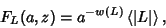(1)

whereis an oriented Link Diagram,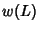is the Writhe of,is the unoriented diagram corresponding to, andis the Bracket Polynomial. It was developed by Kauffman by extending the BLM/Ho Polynomialto two variables, and satisfies(2)

The Kauffman Polynomial is a generalization of the Jones Polynomial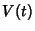since it satisfies(3)

but its relationship to the HOMFLY Polynomial is not well understood. In general, it has more terms than the HOMFLY Polynomial, and is therefore more powerful for discriminating Knots. It is a semi-oriented Polynomial because changing the orientation only changes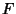by a Power of. In particular, supposeis obtained fromby reversing the orientation of component, then(4)

whereis the Linking Number ofwith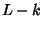(Lickorish and Millett 1988).is unchanged by Mutation.(5)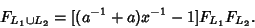(6)

M. B. Thistlethwaite has tabulated the Kauffman 2-variable Polynomial for Knots up to 13 crossings.

References

Lickorish, W. B. R. and Millett, B. R. The New Polynomial Invariants of Knots and Links.'' Math. Mag. 61, 1-23, 1988.

Stoimenow, A. Kauffman Polynomials.'' http://www.informatik.hu-berlin.de/~stoimeno/ptab/k10.html.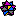Weisstein, E. W. Knots and Links.'' Mathematica notebook Knots.m.